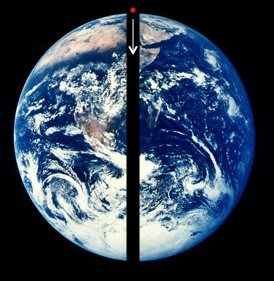# Tunnel across the EarthA straight tunnel is bored through the centre of the earth, A body of mass m is dropped into it. If the motion of body is simple harmonic then find its time period (in second).

Details

1. Radius of the Earth = $6.4 × 10^{6} \text{ m}$
2. $g = 9.8 \text{ ms}^{-2}$ , $\pi = 3.14$
×